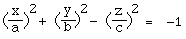Written by Paul Bourke
July 1996

The following are the formulae for some of the more common quadrics, that is, surfaces formed by an equation in x and y of at most degree 2.

Ellipsoid, real (Special case: Sphere)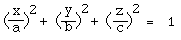Ellipsoid, imaginaryCone, realCone, imaginary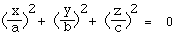Cylinder, real (Elliptic)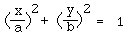Cylinder, imaginary (Elliptic)Cylinder (Hyperbolic)Cylinder (Parabolic)Paraboloid (Elliptic)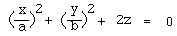Paraboloid (Hyperbolic)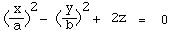Hyperboloid (Single sheet)Hyperboloid (Double sheet)